# Sensing Matrix Optimization for Block-Sparse - Lihi Zelnik

## Transcription

Sensing Matrix Optimization for Block-Sparse - Lihi Zelnik
```1
Sensing Matrix Optimization for Block-Sparse
Decoding
Lihi Zelnik-Manor, Member, IEEE, Kevin Rosenblum, and Yonina C. Eldar, Member, IEEE
Abstract—Recent work has demonstrated that using a carefully
designed sensing matrix rather than a random one, can improve
the performance of compressed sensing. In particular, a welldesigned sensing matrix can reduce the coherence between the
atoms of the equivalent dictionary, and as a consequence, reduce
the reconstruction error. In some applications, the signals of
interest can be well approximated by a union of a small number
of subspaces (e.g., face recognition and motion segmentation).
This implies the existence of a dictionary which leads to blocksparse representations. In this work, we propose a framework
for sensing matrix design that improves the ability of blocksparse approximation techniques to reconstruct and classify
signals. This method is based on minimizing a weighted sum
of the inter-block coherence and the sub-block coherence of the
equivalent dictionary. Our experiments show that the proposed
algorithm significantly improves signal recovery and classification
ability of the Block-OMP algorithm compared to sensing matrix
optimization methods that do not employ block structure.
I. I NTRODUCTION
The framework of compressed sensing aims at recovering
an unknown vector x ∈ RN from an under-determined system
of linear equations y = Ax, where A ∈ RM ×N is a sensing
matrix, and y ∈ RM is an observation vector with M < N .
Since the system is under-determined, x can not be recovered
without additional information. In ,  it was shown that
when x is known to have a sufficiently sparse representation,
and when A is randomly generated, x can be recovered
uniquely with high probability from the measurements y. More
specifically, the assumption is that x can be represented as
x = Dθ for some orthogonal dictionary D ∈ RN ×N , where
θ ∈ RN is sufficiently sparse. The vector x can then be recovered regardless of D and irrespective of the locations of the
nonzero entries of θ. This can be achieved by approximating
the sparsest representation θ using methods such as Basis
Pursuit (BP)  and Orthogonal Matching Pursuit (OMP)
, . In practice, overcomplete dictionaries D ∈ RN ×K
with K ≥ N lead to improved sparse representations and
are better suited for most applications. Therefore, we treat the
more general case of overcomplete dictionaries in this paper.
A simple way to characterize the recovery ability of sparse
approximation algorithms was presented in , using the
coherence between the columns of the equivalent dictionary
E = AD. When the coherence is sufficiently low, OMP and
BP are guaranteed to recover the sparse vector θ. Accordingly,
c 2011 IEEE. Personal use of this material is permitted.
Copyright However, permission to use this material for any other purposes must be
obtained from the IEEE by sending a request to [email protected]
The authors are with the Department of Electrical Engineering, Technion
- Israel Institute of Technology, Haifa, Israel. Email: [email protected],
[email protected], [email protected]
recent work , ,  has demonstrated that designing a
sensing matrix such that the coherence of E is low improves
the ability to recover θ. The proposed methods yield good
results for general sparse vectors.
In some applications, however, the representations have a
unique sparsity structure that can be exploited. Our interest is
in the case of signals that are drawn from a union of a small
number of subspaces , , . This occurs naturally, for
example, in face recognition , , motion segmentation
, multi-band signals , , , measurements of gene
expression levels , and more. For such signals, sorting
the dictionary atoms according to the underlying subspaces
leads to sparse representations which exhibit a block-sparse
structure, i.e., the nonzero coefficients in θ occur in clusters of
varying sizes. Several methods, such as Block-BP (BBP) ,
, , also referred to as Group lasso , , ,
and Block-OMP (BOMP) ,  have been proposed to
take advantage of this block structure in recovering the blocksparse representations θ. Bounds on the recovery performance
were presented in  based on the block restricted isometry
property (RIP), and in  using appropriate coherence measures. In particular, it was shown in  that under conditions
on the inter-block coherence (i.e., the maximal coherence
between two blocks) and the sub-block coherence (i.e., the
maximal coherence between two atoms in the same block)
of the equivalent dictionary E, Block-OMP is guaranteed to
recover the block-sparse vector θ.
In this paper we propose a method for designing a sensing
matrix, assuming that a block-sparsifying dictionary is provided. Our approach improves the recovery ability of blocksparse approximation algorithms by targeting the Gram matrix
of the equivalent dictionary, an approach similar in spirit to
that of , . While  and  targeted minimization of
the coherence between atoms, our method, which will be
referred to as Weighted Coherence Minimization (WCM), aims
at reducing a weighted sum of the inter-block coherence and
the sub-block coherence.
It turns out that the weighted coherence objective is hard to
minimize directly. To derive an efficient algorithm, we use the
bound-optimization method, and replace our objective with an
easier to minimize surrogate function that is updated in each
optimization step . We develop a closed form solution for
minimizing the surrogate function in each step, and prove that
its iterative minimization is guaranteed to converge to a local
solution of the original problem.
Our experiments reveal that minimizing the sub-block coherence is more important than minimizing the inter-block
coherence. By giving more weight to minimizing the sub-block
coherence, the proposed algorithm yields sensing matrices that
2
lead to equivalent dictionaries with nearly orthonormal blocks.
Simulations show that such sensing matrices significantly
improve signal reconstruction and signal classification results
compared to previous approaches that do not employ block
structure.
We begin by reviewing previous work on sensing matrix
design in Section II. In Section III we introduce our definitions
of total inter-block coherence and total sub-block coherence.
We then present the objective for sensing matrix design, and
show that it can be considered as a direct extension of the
one used in  to the case of blocks. We present the WCM
algorithm for minimizing the proposed objective in Section IV
and prove its convergence in Appendix A. We evaluate the
performance of the proposed algorithm and compare it to
previous work in Section V.
Throughout the paper, we denote vectors by lowercase
letters, e.g., x, and matrices by uppercase letters, e.g., A. A0
is the transpose of A. The jth column of the matrix A is Aj ,
and the ith row is Ai . The entry of A in the row with index i
and the column with
index j is Aij . We define the Frobenius
qP
2
norm by kAkF ≡
j kAj k2 , and the lp -norm of a vector
x by kxkp . The l0 -norm kxk0 counts the number of non-zero
entries in x. We denote the identity matrix by I or Is when the
dimension is not clear from the context. The largest eigenvalue
of the positive-semidefinite matrix B 0 B is written as λmax (B).
II. P RIOR WORK ON SENSING MATRIX DESIGN
The goal of sensing matrix design is to construct a sensing
matrix A ∈ RM ×N with M < N that improves the recovery
ability for a given sparsifying dictionary D ∈ RN ×K with
K ≥ N . In other words, A is designed to improve the ability
of sparse approximation algorithms such as BP and OMP to
recover the sparsest representation θ from
y = ADθ = Eθ,
(1)
where E is the equivalent dictionary. In this section we
briefly review the sensing matrix design method introduced by
Duarte-Carvajalino and Sapiro . Their algorithm was shown
to provide significant improvement in reconstruction success.
The motivation to design sensing matrices stems from the
theoretical work of , where it was shown that BP and OMP
succeed in recovering θ when the following condition holds:
1
1
kθk0 ≤
1+
.
(2)
2
µ
Here µ is the coherence defined by:
µ ≡ max
i6=j
|Ei0 Ej |
.
kEi k2 kEj k2
(3)
The smaller µ, the higher the bound on the sparsity of θ.
Since E is overcomplete, and as a consequence not orthogonal,
µ will always be strictly positive. Condition (2) is a worstcase bound and does not reflect the average recovery ability
of sparse approximation methods. However, it does suggest
that recovery may be improved when E is as orthogonal as
possible.
Motivated by these observations, Duarte-Carvajalino and
Sapiro  proposed designing a sensing matrix A by minimizing kE 0 E − Ik2F . This problem can be written as:
min kE 0 E − Ik2F = min kD0 A0 AD − Ik2F .
A
A
(4)
It is important to note that rather than minimizing µ, (4)
minimizes the sum of the squared inner products of all pairs
of atoms in E, referred to as the total coherence µt :
X
µt =
(Ei0 Ej )2 .
(5)
j,i6=j
At the same time, solving (4) keeps the norms of the atoms
close to 1.
While an approximate solution to (4) has already been
presented in , we provide an exact solution that will be of
use in the next sections. To solve (4), we rewrite its objective
using the well-known relation between the Frobenius norm
and the trace, kCk2F = tr(CC 0 ):
kE 0 E − IK k2F =tr(E 0 EE 0 E − 2E 0 E + IK )
=tr(EE 0 EE 0 − 2EE 0 + IM ) + (K − M )
=kEE 0 − IM k2F + (K − M )
=kADD0 A0 − IM k2F + (K − M ).
(6)
Since the first term in (6) is always positive, the objective of
(4) is lower bounded by kE 0 E − Ik2F ≥ K − M .
From (6) it follows that minimizing (4) is equivalent to the
minimization of kADD0 A0 −IM k2F . A solution to this problem
can be achieved in closed form as follows. Let U ΛU 0 be the
eigenvalue decomposition of DD0 , and let ΓM ×N = AU Λ1/2 .
Then, (4) is equivalent to:
min kΓΓ0 − Ik2F .
A
(7)
This problem is solved by choosing Γ to be any matrix with
orthonormal rows, such as Γ = [IM 0], leading to ΓΓ0 = I.
The optimal sensing matrix is then given by A = ΓΛ−1/2 U 0 .
Here, and throughout the paper, we assume that D has full row
rank, guaranteeing that Λ is invertible. Note that the global
minimum of the objective in (4) equals K − M . The benefits
of using such a sensing matrix were shown empirically in .
III. S ENSING MATRIX DESIGN FOR BLOCK - SPARSE
DECODING
The design of a sensing matrix according to  does not
take advantage of block structure in the sparse representations
of the data. In this section we formulate the problem of sensing
matrix design for block-sparse decoding. We first introduce the
basic concepts of block-sparsity, and then present an objective
which can be seen as an extension of (4) to the case of blocksparsity.
A. Block-sparse decoding
The framework of block-sparse decoding aims at recovering
an unknown vector x ∈ RN from an under-determined system
of linear equations y = Ax, where A ∈ RM ×N is a sensing
matrix, and y ∈ RM is an observation vector with M < N .
3
The difference with sparse recovery lies in the assumption that
x has a sufficiently block-sparse representation θ ∈ RN with
respect to some block-sparsifying dictionary D ∈ RN ×N . The
vector x can then be recovered by approximating the blocksparsest representation corresponding to the measurements y
using methods such as Block-BP (BBP) , ,  and
Block-OMP (BOMP) , .
A block-sparsifying dictionary D is a dictionary whose
atoms are sorted in blocks which enable block-sparse representations for a set of signals. We can represent D as a
concatenation of B column-blocks D[j] of size N × sj , where
sj is the number of atoms belonging to the jth block:
D = [D D . . . D[B]].
Similarly, we view the representation θ as a concatenation of
B blocks θ[j] of length sj :
θ = [θ θ . . . θ[B]]0 .
We say that a representation θ is k-block-sparse if its nonzero
values are concentrated in k blocks only. This is denoted by
kθk2,0 ≤ k, where
kθk2,0 =
B
X
I(kθ[j]k2 > 0).
in E. The sub-block coherence ν describes the local properties
of the dictionary, by measuring the cosine of the minimal angle
between two atoms in the same block in E. Note, that when
s = 1, (8) reduces to the bound in the sparse case (2). The
term µ−1
B in (8) suggests that µB needs to be reduced in order
to loosen the bound. On the other hand, the term −(s − 1) µνB
implies that the ratio µνB should be small. This leads to a
trade-off between minimizing µB and minimizing ν to loosen
the bound, which is reflected in the sensing matrix design
objective presented later in this section.
Condition (8) is a worst case bound and does not represent
the average recovery ability of block-sparse approximation
methods. It does suggest, however, that in order to improve
the average recovery, all pairs of blocks in E should be as
orthogonal as possible and also all pairs of atoms within each
block should be as orthogonal as possible. Inspired by ,
rather than minimizing the inter-block coherence µB and the
sub-block coherence ν, we aim at minimizing the total interblock coherence µtB and the total sub-block coherence ν t of
the equivalent dictionary E. We define the total inter-block
coherence as
B X
X
kG[i, j]k2F ,
(9)
µtB =
j=1 i6=j
j=1
and the total sub-block coherence by
The indicator function I(·) counts the number of blocks in θ
with nonzero Euclidean norm.
νt =
B
X
j=1
B. Problem definition
N ×K
For a given block-sparsifying dictionary D ∈ R
with
K ≥ N , we wish to design a sensing matrix A ∈ RM ×N that
improves the recovery ability of block-sparse approximation
algorithms. Note that we allow D to be overcomplete.
A performance bound on the recovery success of blocksparse signals has been developed in  for the case of a dictionary D with blocks of a fixed size s (i.e., si = sj = s) and
an equivalent dictionary E = AD with normalized columns.
The bound is a function of the Gram matrix G ∈ RK×K of the
equivalent dictionary, defined as E 0 E. The (i, j)th block of G,
E[i]0 E[j], is denoted by G[i, j] ∈ Rsi ×sj . The (i, j)th block of
any other K×K matrix will be denoted similarly. It was shown
in  that BBP and BOMP succeed in recovering the block
sparsest representation θ corresponding to the measurements
y = Eθ when the following condition holds:
ν
1
µ−1
+
s
−
(s
−
1)
.
(8)
kθk2,0 <
B
2s
µB
Here
µB ≡ max
j,i6=j
1p
λmax (G[i, j]0 G[i, j])
s
is the inter-block coherence and
ν ≡ max max |(G[j, j])m
n|
j
n,m6=n
is the sub-block coherence. The inter-block coherence µB is
a generalization of the coherence µ, and describes the global
properties of the equivalent dictionary. More specifically, µB
measures the cosine of the minimal angle between two blocks
kG[j, j]k2F −
K
X
2
(Gm
m) ,
(10)
m=1
where Gm
m are the diagonal entries of G. The total inter-block
coherence µtB equals the sum of the squared entries in G
belonging to different blocks (the green entries in Fig. 1).
Since this is the sum of Frobenius norms, µtB also equals
the sum of the squared singular values of the cross-correlation
blocks in G. When E is normalized, µtB is equivalent to the
sum of the squared cosines of all the principal angles between
all pairs of different blocks. The total sub-block coherence ν t
measures the sum of the squared off-diagonal entries belonging
to the same block (the red entries in Fig. 1). When E is
normalized, ν t equals the sum of the squared cosines of all
the angles between atoms within the same block. Note that
when the size of the blocks equals one, we get ν t = 0.
Alternatively, one could define the total inter-block coherence as the sum of the squared spectral norms (i.e., the
largest singular values) of the cross-correlation blocks in G,
and the total sub-block coherence as the sum of the squared
maximal off-diagonal entries of the auto-correlation blocks in
G. These definitions are closer to the ones used in condition
(8). The WCM algorithm presented in the next section can be
slightly modified in order to minimize those measures as well.
However, besides the increased complexity of the algorithm,
the results appear to be inferior compared to minimizing the
definitions (9) and (10) of µtB and ν t . This can be explained
by the fact that maximizing only the smallest principal angle
between pairs of different blocks in E and maximizing the
smallest angle between atoms within the same block, creates
a bulk of relatively high singular values and coherence values.
While this may improve the worst-case bound in (8), it does
4
Fig. 1. A graphical depiction of the Gram matrix
G of an equivalent dictionary E with 6 blocks of
size 3. The entries belonging to different blocks are
in green, the off-diagonal entries belonging to the
same block are in red, and the diagonal entries are
in yellow.
(a)
When minimizing the total inter-block coherence and the
total sub-block coherence, we need to verify that the columns
of E are normalized, to avoid the tendency of columns with
small norm values to be underused. Rather than enforcing
normalization strongly, we penalize for columns with norms
that deviate from 1 by defining the normalization penalty η:
K
X
2
(Gm
m − 1) .
(11)
m=1
This penalty η measures the sum of the squared distances
between the diagonal entries in G (the yellow entries in Fig.
1) and 1.
While  did not deal with the block-sparse case, it is
straightforward to see that solving (4) is equivalent to minimizing the sum of the normalization penalty, the total inter-block
coherence and the total sub-block coherence:
kE 0 E − Ik2F =
B X
X
kE[i]0 E[j]k2F +
=
kG[i, j]k2F +
j=1 i6=j
=
B X
X
=η +
B
X
kG[j, j] − Ik2F
j=1
kG[i, j]k2F +
j=1 i6=j
−
kE[j]0 E[j] − Ik2F
j=1
j=1 i6=j
B X
X
B
X
K
X
B
X
kG[j, j]k2F
j=1
2
(Gm
m) +
m=1
µtB +
K
X
2
(Gm
m − 1)
m=1
t
ν .
We have shown in the previous section that the objective in
(4) is bounded below by K − M . Therefore,
η + µtB + ν t ≥ K − M.
(c)
Fig. 2. Examples of the absolute value of the Gram matrix of an equivalent dictionary for
α = 0.01 (a), α = 0.5 (b) and α = 0.99 (c), where the sensing matrix of size 12 × 18 was
found by solving (13) given a randomly selected square dictionary composed of 6 blocks of size
3. The sub-block entries are highlighted by red squares.
not necessarily improve the average recovery ability of blocksparse approximation methods.
η=
(b)
(12)
This bound implies a trade-off, and as a consequence, one
cannot minimize η, µtB and ν t freely. Instead, we propose
designing a sensing matrix that minimizes the normalization
penalty and a weighted sum of the total inter-block coherence
and the total sub-block coherence:
1
A = arg min η + (1 − α)µtB + αν t ,
(13)
A 2
where 0 < α < 1 is a parameter controlling the weight
given to the total inter-block coherence and the total sub-block
coherence. Note that alternative objectives can be formulated.
For example, one could add an additional weighting parameter
to the normalization penalty term. While this would allow us
to better control the normalization of the atoms in E, we prefer
to deal with a single parameter only.
When α < 21 , more weight is given to minimizing µtB ,
and therefore solving (13) leads to lower total inter-block
coherence, which is made possible by aligning the atoms
within each block (Fig. 2(a)). On the other hand, choosing
α > 12 gives more weight to minimizing ν t . In this case,
solving (13) leads to more orthonormal blocks in E at the
expense of higher µtB (Fig. 2(c)). Finally, setting α = 12 in
(13) gives equal weights to µtB , ν t and η, and reduces it to
(4) (Fig. 2(b)). Therefore, the objective becomes independent
of the block structure, which makes α = 12 the correct
choice when the signals do not have an underlying block
structure. Choosing to ignore the block structure leads to the
same conclusion. When an underlying block structure exists,
we need to select a value for α. We do that via empirical
evaluation in Section V.
In the next section we present an iterative algorithm that
converges to a local solution of (13). Although we can only
guarantee a local minimum, in our simulations we have noticed
that independent executions of the algorithm tend to reach the
same solution. Our empirical observations are demonstrated in
the histograms in Fig. 3 (square dictionary) and Fig. 4 (a highly
overcomplete dictionary), for both α = 0.01 and α = 0.99.
IV. W EIGHTED C OHERENCE M INIMIZATION
In this section, we present the Weighted Coherence Minimization (WCM) algorithm for minimizing (13), based on the
bound-optimization method . This algorithm substitutes
the original objective with an easier to minimize surrogate
objective that is updated in each optimization step. After
defining a surrogate function and showing it can be minimized
in closed form, we prove that its iterative minimization is
guaranteed to converge to a local solution of the original
problem.
A. The Weighted Coherence Minimization Algorithm
To obtain a surrogate function we rewrite the objective of
(13), which we denote by f (G), as a function of the Gram
5
Objective value for α=0.01
Objective value for α=0.99
50
100
45
90
40
80
35
70
Objective value for α=0.01
25
20
30
10
20
5
10
0.1
0.12
0.14
(a)
0.16
0.18
0
0.0891
90
45
80
40
70
35
60
30
50
40
15
0
0.08
50
50
Frequency
60
Frequency
30
Objective value for α=0.99
100
0.0891
0.0891
25
40
20
30
15
20
10
10
5
0.0891
(b)
0
32.35
32.4
32.45
32.5
(a)
Fig. 3. Histograms of the objective values obtained when solving
(13) 100 times with α = 0.01 (a) and α = 0.99 (b), for a given
randomly generated square dictionary composed of 6 blocks of size
3. The sensing matrices of size 12 × 18 are initialized as matrices
with random entries. Note that the distribution is insignificant in (b),
indicating that all the local minimum that our algorithm detected had
the same objective value.
matrix of the equivalent dictionary G = D0 A0 AD:
1
f (G) ≡ η(G) + (1 − α)µtB (G) + αν t (G)
2
1
= kuη (G)k2F + (1 − α)kuµ (G)k2F + αkuν (G)k2F ,
2
where the matrix operators uµ , uν and uη are defined as:
G[i, j]m
m
n − 1, i = j, m = n;
uη (G)[i, j]n =
,
0,
else
G[i, j]m
n , i 6= j;
uµ (G)[i, j]m
,
n =
0,
else
G[i, j]m
n , i = j, m 6= n;
,
uν (G)[i, j]m
n =
0,
else
with G[i, j]m
n denoting the (m, n)th entry of G[i, j]. This
equation follows directly from the definitions of η, µtB and
ν t . We can now write:
1
f (G) = kG − hη (G)k2F + (1 − α)kG − hµ (G)k2F
2
+αkG − hν (G)k2F , (14)
where the matrix operators hµ , hν and hη are defined as:
1,
i = j, m = n;
m
hη (G)[i, j]n =
,
G[i, j]m
,
else
n
0,
i 6= j;
hµ (G)[i, j]m
,
n =
G[i, j]m
n , else
0,
i = j, m 6= n;
hν (G)[i, j]m
=
.
n
G[i, j]m
n , else
0
3.7
3.72
3.74
3.76
3.78
3.8
(b)
Fig. 4. Histograms of the objective values obtained when solving (13)
100 times with α = 0.01 (a) and α = 0.99 (b), for a given randomly
generated overcomplete dictionary composed of 24 blocks of size 3.
The sensing matrices of size 12 × 18 are initialized as matrices with
random entries.
0
where G(n) = D0 A(n) A(n) D is the Gram matrix of the
equivalent dictionary from the previous iteration. In Appendix
B, we prove that g(G, G(n) ) satisfies the conditions of a surrogate objective for the bound-optimization method. Therefore,
iteratively minimizing g(G, G(n) ) is guaranteed to converge
to a local minimum of the original objective f (G), i.e., solve
(13).
The following proposition describes the closed form solution to minimizing g(G, G(n) ) at each iteration.
Proposition 1: The function g(G, G(n) ) is minimized by
choosing
1/2 0
A(n+1) = ∆M VM
Λ−1/2 U 0 ,
where U ΛU 0 is the eigenvalue decomposition of DD0 , ∆M
and VM are the top M eigenvalues and the corresponding M
eigenvectors of Λ−1/2 U 0 Dht (G(n) )D0 U Λ−1/2 , and:
2 1
ht (·) ≡
hη (·) + (1 − α)hµ (·) + αhν (·) .
(16)
3 2
Proof: See Appendix B.
A summary of the proposed WCM algorithm is given below.
The computation complexity of this algorithm is O(N 3 ).
This is since every iteration includes finding the M principal
components of an N × N matrix (the sensing matrix A is in
M × N ), i.e., SVD of complexity N 3 .
V. E XPERIMENTS
In this section, we evaluate the contribution of the proposed
sensing matrix design framework empirically. We compare
the recovery and classification abilities of BOMP , 
when using sensing matrices designed by our methods to the
outcome of (4), which will be referred to as “Duarte-Sapiro”
(DS) .
Based on (14), we define a surrogate objective g(G, G(n) )
at the nth iteration as:
A. Experiments on synthetic data
1
g(G, G(n) ) ≡ kG − hη (G(n) )k2F + (1 − α)kG − hµ (G(n) )k2F
2
For each simulation, we repeat the following procedure
+αkG − hν (G(n) )k2F , 100 times. We randomly generate a dictionary DN ×K with
(15) normally distributed entries and normalize its columns. In
6
Algorithm 1 Weighted Coherence Minimization
Task: Solve for a given block-sparsifying dictionary DN ×K :
1
A = arg min η + (1 − α)µtB + αν t ,
A 2
where A ∈ RM ×N .
Initialization: Calculate the eigenvalue decomposition of
DD0 = U ΛU 0 . Set A(0) as the outcome of (4), i.e., A(0) =
[IM 0]Λ−1/2 U 0 , and n = 0.
Repeat until convergence:
0
1) Set G(n) = D0 A(n) A(n) D.
(n)
2) Calculate ht (G ) as in (16).
3) Find the top M eigenvalues ∆M and the corresponding
M eigenvectors VM of Λ−1/2 U 0 Dht (G(n) )D0 U Λ−1/2 .
1/2 0
4) Set A(n+1) = ∆M VM
Λ−1/2 U 0 .
5) n = n + 1.
order to evaluate WCM on structured dictionaries as well,
we repeat the simulations using a dictionary containing N
randomly selected rows of the K × K Discrete Cosine Transform (DCT) matrix. The dictionary is divided into K/s blocks
of size s. We then generate L = 1000 test signals X of
dimension K that have k-block-sparse representations Θ with
respect to D. The generating blocks are chosen randomly
and independently and the coefficients are i.i.d. uniformly
distributed. We compare three options for designing AM ×N :
(i) random, (ii) the outcome of DS and (iii) the proposed
WCM which is initialized as the outcome of DS. Having found
A we calculate the equivalent dictionary E = AD and the
measurements Y = AX. Next, we obtain the block-sparsest
representations of the measurements, Θ̂, by applying BOMP
with a fixed number of k nonzero blocks.
We use two measures to evaluate the success of the simulations based on their outputs A and Θ̂:
• The percentage of recognized generating subspaces of X
0
(i.e., successful classification): r = kΘ̂Θk
Lks
where denotes element-wise multiplication.
kX−D Θ̂kF
• The normalized representation error e =
kXkF
Tuning α: To evaluate the performance of the WCM
algorithm as a function of α, we choose s = 3, N = 60
and K = 2N = 120. We repeat the experiment for both
types of dictionaries, and for k = 1 (Fig. 5(a),5(b)), k = 2
(Fig. 6(a),6(b)) and k = 3 (Fig. 7(a),7(b)) nonzero blocks, with
respectively M = 6, M = 14 and M = 20 measurements. To
show that the results remain consistent for higher values of k,
we add an experiment with k = 6, M = 35, N = 180 and
K = 2N = 360 (Fig. 8(a),8(b)). We compare the obtained
results to randomly set sensing matrices and to the outputs of
DS , based on the normalized representation error e, the
classification success r, and the ratio between the total subblock coherence and the total inter-block coherence ν t /µtB .
The results indicate that WCM and DS coincide at α = 0.5
for all the three measures, as expected. Note that for α < 0.5
we get that ν t /µtB is high, e is high and r is low. On the
other hand, when α > 0.5, i.e., when giving more weight to
ν t and less to µtB , the signal reconstruction as well as the
signal classification are improved compared to DS. This is in
line with our earlier observation based on the bound in (8) that
the ratio µνB should be small. Indeed our experiments show
that when α > 0.5 we get that ν t /µtB is low. The simulation
results show a dramatic change in performance around the
value α = 0.5. This is since at α = 0.5 the importance of ν t
becomes higher than that of µtB , which changes the behavior
of the algorithm.
While the improvement for k = 1 is more significant, it
is maintained for higher values of k as well. Remarkably,
for structured dictionaries and for higher values of k, we
see that α < 0.5 leads to an improvement of r. However,
e is compromised in this case. We can conclude that when
designing sensing matrices for block sparse decoding, the best
results are obtained by choosing α close enough to 1. In other
words, the best recovery results are obtained when the equivalent dictionary has nearly orthonormal blocks. This holds
for dictionaries containing normally distributed entries as well
as for dictionaries containing randomly selected rows of the
DCT matrix. When the dictionary is over-complete the blocks
cannot be truly orthonormal. Based on these observations we
conclude that α ≈ 1 is the best value. In our experiments
on real data (next section) setting α = 1 led to the same
results as values close to 1. However, we did not want to
completely ignore the inter-block coherence thus we typically
set α = 0.99.
As mentioned before, WCM converges but could get trapped
in local minima. As was shown in Fig. 3(b), we observed
empirically that for α > 0.5, every local minimum reached had
the same objective value. We observed this empirical behavior
also in the exhaustive experiments presented in this section.
This means that the WCM algorithm converges consistently
to the same solution of (13) when α > 0.5, for all the
experiments presented in this section. We emphasize however,
that this may not be the case for other sets of parameters.
Dictionary dimension: Fig. 9(a) and Fig. 9(b) show that
when using WCM with α = 0.99 on dictionaries with
normally distributed entries and on structured dictionaries,
the improvement in signal recovery is maintained for a wide
range of K, starting from square dictionaries, i.e. K = N ,
to highly over-complete dictionaries. For this experiment, we
chose s = 3, N = 60, k = 2 and M = 14. We note that for
both types of dictionaries, the improvement of WCM over DS
increases as the dictionary becomes more over-complete.
Varying block sizes: Finally, we show that WCM improves
the results of block-sparse decoding for dictionaries with
blocks of varying sizes as well. The generated dictionaries
contain 15 blocks of size 4 and 20 blocks of size 3, with
N = 60 and K = 2N = 120. In this example, we set k = 2
and M = 14. The results are shown as a function of α in
Fig. 10(a) for dictionaries with normally distributed entries
and in Fig. 10(b) for structured dictionaries.
B. Experiments on real image data
To evaluate the applicability of the proposed approach in
practical scenarios we further perform experiments on real
image data. Similar to  we experimented with compression
7
100
100
80
80
90
90
85
85
80
WCM
DS
Random
40
20
0.2
0.4
α
0.6
20
0.8
1.5
0.2
0.4
α
0.6
75
70
WCM
DS
Random
40
80
75
r
r
60
r
r
60
WCM
DS
Random
65
60
0.8
0.2
0.4
α
0.6
65
0.8
1.5
0.5
α
0.6
0
0.8
0
0.2
0.4
α
0.6
0.8
10
−2
0.4
α
0.6
0.4
0.8
10
−5
10
0.4
0.6
0.8
−10
−8
0.4
α
0.6
10
0.8
−15
−15
0.2
(a)
0.4
α
0.6
10
0.8
0.2
(b)
75
70
0.6
10
0.8
(b)
65
70
60
65
55
70
WCM
DS
Random
65
0.2
0.4
α
0.6
WCM
DS
Random
65
60
0.8
0.4
α
0.6
WCM
DS
Random
50
45
0.8
0.2
0.4
α
0.6
50
0.8
α
0.6
0.45
0.8
0
10
−5
10
0.2
0.4
α
0.6
0.8
0.2
(a)
α
0.6
0.8
0.6
10
0.7
0.8
10
−5
10
α
0.6
0.8
(b)
Fig. 6. Simulation results of sensing matrix design using the WCM algorithm
with k = 2 and M = 14. The graphs show the normalized representation
error e, the classification success r, and the ratio between the total sub-block
coherence and the total inter-block coherence ν t /µtB as a function of α. In
(a) the dictionary contains normally distributed entries, and in (b) randomly
selected rows of the DCT matrix.
0.2
0.4
α
0.8
0.6
0.8
−10
10
−15
−15
0.4
0.4
−5
−10
0.2
0.2
0
0
10
−15
0.4
α
νW μW%
−10
0.2
0.4
10
10
−15
0.6
0.75
10
νt /μtB
t
t
ν /μB
−10
10
0.8
0.8
0.7
−5
10
0.8
0.65
0
10
0.6
0.85
0.75
0.5
0.4
α
e
0.6
e
e
0.85
0.55
0.2
0.4
0.9
0.9
0.65
0.5
0.2
0.95
0.95
0.7
0.6
WCM
DS
Random
55
0.75
0.7
60
νt /μtB
0.8
0.2
α
Fig. 7. Simulation results of sensing matrix design using the WCM algorithm
with k = 3 and M = 20. The graphs show the normalized representation
error e, the classification success r, and the ratio between the total sub-block
coherence and the total inter-block coherence ν t /µtB as a function of α. In
(a) the dictionary contains normally distributed entries, and in (b) randomly
selected rows of the DCT matrix.
r
75
r
80
r
85
80
α
(a)
Fig. 5. Simulation results of sensing matrix design using the WCM algorithm
with k = 1 and M = 6. The graphs show the normalized representation
error e, the classification success r, and the ratio between the total sub-block
coherence and the total inter-block coherence ν t /µtB as a function of α. In
(a) the dictionary contains normally distributed entries, and in (b) randomly
selected rows of the DCT matrix.
85
0.4
r
0.2
10
10
−6
10
−8
e
0.8
νt /μtB
νW μW%
νt /μtB
νt /μtB
−4
10
−10
−6
10
10
0.2
α
−5
10
0.4
0.4
0
0
10
10
−4
10
60
0.2
−2
10
10
0.6
0.45
0.2
0
10
0.8
0.5
0.4
0.4
0.6
0.55
0.45
0.5
0.2
α
e
e
e
e
0.55
0.35
0
0.4
0.6
0.6
1
0.5
0.2
0.65
0.65
1
WCM
DS
Random
70
10
0.2
0.4
(a)
α
0.6
0.8
10
α
(b)
Fig. 8. Simulation results of sensing matrix design using the WCM algorithm
with k = 6 and M = 35. The graphs show the normalized representation
error e, the classification success r, and the ratio between the total sub-block
coherence and the total inter-block coherence ν t /µtB as a function of α. In
(a) the dictionary contains normally distributed entries, and in (b) randomly
selected rows of the DCT matrix.
8
100
100
WCM(α=0.99)
DS
Random
90
80
Random
DS
WCM
0.25
Random
DS
WCM
0.25
0.2
0.2
0.15
0.15
e
e
80
70
70
r
r
WCM(α=0.99)
DS
Random
90
60
0.1
0.1
0.05
0.05
60
50
50
40
100
150
200
250
300
0
40
100
K
150
200
250
300
K
0.5
0.6
0.7
0.8
alpha
0.9
1
0
0.5
0.6
k=1
0.7
0.8
alpha
0.9
1
k=2
Fig. 12. Repeating the experiment of Fig. 11 for varying values of α. When
α > 0.5 WCM results in lower errors compared to DS and random A.
0.9
0.8
0.8
0.7
e
e
0.7
0.6
0.6
0.5
0.4
0.5
0.3
0.4
100
150
200
250
300
100
K
150
200
250
300
K
(a)
(b)
Fig. 9. Simulation results of sensing matrix design using the WCM algorithm
with k = 2 and M = 14. The graphs show the normalized representation
error e and the classification success r as a function of K. In (a) the dictionary
contains normally distributed entries, and in (b) randomly selected rows of
the DCT matrix.
80
80
75
75
r
70
r
70
65
60
55
65
WCM
DS
Random
0.2
60
0.4
α
0.6
55
0.8
0.9
0.2
0.4
α
0.6
0.8
0.6
0.8
0.6
0.8
0.9
0.8
0.8
e
e
0.7
0.6
0.7
0.6
0.5
0.4
WCM
DS
Random
0.2
0.4
α
0.6
0.5
0.8
0
0.2
0.4
0.2
0.4
α
0
10
10
−5
νt /μtB
νt /μtB
10
−5
10
−10
10
−10
10
−15
0.2
0.4
(a)
α
0.6
0.8
10
α
(b)
Fig. 10.
Simulation results of sensing matrix design using the WCM
algorithm on a dictionary containing 15 blocks of size 4 and 20 blocks of size
3, with k = 2 and M = 14. The graphs show the normalized representation
error e, the classification success r, and the ratio between the total sub-block
coherence and the total inter-block coherence ν t /µtB as a function of α. In
(a) the dictionary contains normally distributed entries, and in (b) randomly
selected rows of the DCT matrix.
of image patches. We begin by hand-crafting a block-sparse
dictionary D. We select B = 50 random locations in a given
training image (Fig. 11(a)) and extract around each location 9
overlapping patches of size 7 × 7, which are reshaped into 49
dimensional column vectors. We then fit a linear subspace of
dimension s = 6 to each group of overlapping patches. The
computed 50 subspaces are used to construct our dictionary
D (i.e., K = 300).
We then take 500 different testing images (see an example
in Fig. 11(b)) and extract from each image all the non-
overlapping patches of size 7 × 7, which are reshaped into
49 dimensional column vectors. They are compressed into
M = 15 dimensions using a sensing matrix AM ×N . Three
sensing matrices are tested: (i) random, (ii) designed according
to DS  and (iii) designed according to our WCM algorithm
with α = 0.99. Next, we obtain the block-sparse representations Θ of the signals by applying BOMP with a fixed
number of k = 2 non-zero blocks. The sparse representations
Θ are used to recover the original signals of size 49. We then
compute the mean representation over all image patches. For
presentation purposes, we further reshape the estimated signals
into 7 × 7 patches and reconstruct the image.
The above procedure was repeated 10 times, for 10 different
dictionaries (each obtained using a different random selection
of the training subspaces). Fig. 11(c) presents the mean
representation error over the 10 trials for each of the 500
testing images. WCM consistently leads to smaller errors.
Next, we repeat the same experiment but replacing the
hand-crafted dictionary with one trained using the approach
proposed in . To train the dictionary we extract all 7 × 7
patches from the training image (total of 294). We then use
SAC+BKSVD (see ) to train the dictionary D and recover
the block structure. The rest of the experiment remains the
same. As shown in Fig. 11(g)-(i), using the trained dictionary
instead of the designed one has little effect on the results.
Finally, we further evaluate the results as a function of α.
Fig. 12 demonstrates that the results on image compression.
When α > 0.5 the reconstruction error is smaller for WCM
than for DS or for a random A. Note, that this is true for both
k = 1 and k = 2, however, the improvement for k = 2 is
more significant.
VI. C ONCLUSIONS
In this paper, we proposed a framework for the design of a
sensing matrix, assuming that a block-sparsifying dictionary is
provided. We minimize a weighted sum of the total inter-block
coherence and the total sub-block coherence, while attempting
to keep the atoms in the equivalent dictionary as normalized as
possible (see (13)). This objective can be seen as an intuitive
extension of (4) to the case of blocks.
While it might be possible to derive a closed form solution
to (13), we have presented the Weighted Coherence Minimization algorithm, an elegant iterative solution which is based on
the bound-optimization method. In this method, the original
objective is replaced with an easier to solve surrogate objective
9
(a) Training image
1
0.8
(b) An example query image
(c) Random A
(e) A trained by DS
D designed
(f) A trained by WCM
D designed
(h) A trained by DS
D trained by 
(i) A trained by WCM
D trained by 
Random
DS
WCM
e
0.6
0.4
0.2
0
100
200
300
Image index
400
500
(d) Representation error,
D designed
1
0.8
Random
DS
WCM
e
0.6
0.4
0.2
0
100
200
300
Image index
400
(g) Representation error,
D trained by 
500
Fig. 11. Image compression experiment. (a) An image used to train sensing matrices using DS and WCM. (b) An example of a query image (500 total)
that was decomposed into 7 × 7 non-overlapping patches which were compressed into 15 dimensions using each of the sensing matrices. We then use BOMP
to recover the sparse representation of the original patches and reconstruct them. (d,g) Representation errors for each of the 7 different query images. WCM
leads to smallest errors on almost all the images. (c,e,f,h,i) The obtained reconstructions.
in each step. This algorithm eventually converges to a local
solution of (13).
Simulations have shown that the best results are obtained when minimizing mostly the total sub-block coherence.
This leads to equivalent dictionaries with nearly orthonormal
blocks, at the price of a slightly increased total inter-block
coherence. The obtained sensing matrix outperforms the one
obtained when using the DS algorithm  to solve (4). This
improvement manifests itself in lower signal reconstruction
errors and higher rates of successful signal classification.
When giving equal weight to the total inter-block coherence
and to the total sub-block coherence, the results are identical to
solving (4). Moreover, both objectives coincide for this specific
choice of α, which ignores the existence of a block structure
in the sparse representations of the signal data.
A PPENDIX A
P ROOF OF CONVERGENCE
The surrogate function g(G, G(n) ) has been chosen in such
a way as to bound the original objective f (G) from above for
every G, and to coincide at G = G(n) . Minimizing g(G, G(n) )
will then necessarily decrease the value of f (G):
min g(G, G(n) ) ≤ g(G(n) , G(n) ) = f (G(n) ),
G
f (G(n+1) ) ≤ g(G(n+1) , G(n) ) = min g(G, G(n) ).
G
Formally, according to , the sequence of solutions generated by iteratively solving
G(n+1) = arg min g(G, G(n) )
G
(17)
is guaranteed to converge to a local minimum of the original objective f (G) when the surrogate objective g(G, G(n) )
satisfies the following three constraints:
10
When substituting G = G(n) we obtain:
1) Equality at G = G(n) :
g(G(n) , G(n) ) = f (G(n) ).
2) Upper-bounding the original function:
g(G, G(n) ) ≥ f (G), ∀G.
3) Equal gradient at G = G(n) :
∇g(G, G(n) )|G=G(n) = ∇f (G)|G=G(n)
1
= 2( uη (G(n) ) + (1 − α)uµ (G(n) ) + αuν (G(n) )).
2
Therefore, the gradients of both objectives coincide at G =
G(n) . This completes the convergence proof.
∇g(G, G(n) )|G=G(n) = ∇f (G)|G=G(n) .
A PPENDIX B
P ROOF OF P ROPOSITION 1
We next prove that the three conditions hold.
Proof: Equality at G = G(n) : This follows from the
definition of g(G, G(n) ).
Upper-bounding the original function: Let us rewrite both
functions g(G, G(n) ) and f (G) using the definition of the
Frobenius norm:
g(G, G(n) ) =
X X 1
i,j m,n
2
2
((G − hη (G(n) ))[i, j]m
n)
+ (1 − α)((G − hµ (G
2
))[i, j]m
n)
i
2
+α((G − hν (G(n) ))[i, j]m
,
n)
and
i,j m,n
2
min g(G, ·) =
A
3 0
0 1
min tr
G G − 2G
hη (·) + (1 − α)hµ (·) + αhν (·)
A
2
2
= min tr(E 0 EE 0 E − 2E 0 Eht (·))
A
= min tr(EE 0 EE 0 − 2Eht (·)E 0 )
A
(n)
f (G) =
X X 1
Proof: In order to minimize g(G, G(n) ), we rewrite the
problem in an alternative form:
= min tr(ADD0 A0 ADD0 A0 − 2ADht (·)D0 A0 ),
A
(18)
where ht (·) is defined in (16). Let U ΛU 0 be the eigenvalue
decomposition of DD0 and define ΓM ×N = AU Λ1/2 . Substituting into (18) yields:
min g(G, ·) =
A
2
m 2
(uη (G)[i, j]m
n ) + (1 − α)(uµ (G)[i, j]n )
2
+α(uν (G)[i, j]m
n)
.
The following observations prove that each of the terms in
g(G, G(n) ) is larger than or equal to its counterpart in f (G),
and therefore g(G, G(n) ) ≥ f (G):
G[i, j]m
m
n − 1, i = j, m = n;
uη (G)[i, j]n =
0,
else.
m
G[i, j]n − 1, i = j, m = n;
(G − hη (G(n) ))[i, j]m
n =
(G − G(n) )[i, j]m
n , else.
m
G[i, j]n , i 6= j;
uµ (G)[i, j]m
n =
0,
else.
m
G[i,
j]
,
i 6= j;
n
(G − hµ (G(n) ))[i, j]m
n =
(n)
m
(G − G )[i, j]n , else.
G[i,
j]m
n , i = j, m 6= n;
uν (G)[i, j]m
n =
0,
else.
m
G[i, j]n , i = j, m 6= n;
(G − hν (G(n) ))[i, j]m
n =
(G − G(n) )[i, j]m
n , else.
Equal gradient at G = G(n) : We calculate the gradient of
g(G, G(n) ) and f (G):
∇g(G, G(n) ) =
1
2 (G − hη (G(n) )) + (1 − α)(G − hµ (G(n) ))
2
i
+α(G − hν (G(n) )) ,
1
∇f (G) = 2 uη (G) + (1 − α)uµ (G) + αuν (G) .
2
min tr(ΓΓ0 ΓΓ0 − 2ΓΛ−1/2 U 0 Dht (·)D0 U Λ−1/2 Γ0 )
A
= min kΓ0 Γ − h̃t (·)k2F ,
A
(19)
where h̃t (·) ≡ Λ−1/2 U 0 Dht (·)D0 U Λ−1/2 . According to (19),
the surrogate objective g(G, G(n) ) can be minimized in closed
form by finding the top M components of h̃t (G(n) ). Let
∆M be the top M eigenvalues of h̃t (G(n) ) and VM the
corresponding M eigenvectors. Then, (19) is solved by setting
1/2 0
. Note that this solution is not unique, since
Γ = ∆M VM
Γ can be multiplied on the left by any unitary matrix. This,
however, does not affect the WCM algorithm since we only
care about updating the Gram matrix G(n+1) , which is not
influenced by the multiplication of A(n+1) on the left by a
unitary matrix. Finally, the optimal sensing matrix is given by
1/2 0
A(n+1) = ΓΛ−1/2 U 0 = ∆M VM
Λ−1/2 U 0 .
ACKNOWLEDGEMENTS
The research of Lihi Zelnik-Manor is supported by Marie
Curie IRG-208529 and by the Ollendorf foundation. The work
of Yonina Eldar was supported in part by a Magneton grant
from the Israel Ministry of Industry and Trade, and by the
Israel Science Foundation under Grant 170/10.
R EFERENCES
 E. Candes, J. Romberg, and T. Tao, “Robust uncertainty principles: Exact
signal reconstruction from highly incomplete frequency information,”
IEEE Trans. Inform. Theory, vol. 52, pp. 489–509, Feb. 2006.
 D. Donoho, “Compressed sensing,” IEEE Trans. Inform. Theory, vol. 52,
no. 4, pp. 1289–1306, Apr. 2006.
 S. S. Chen, D. L. Donoho, and M. A. Saunders, “Atomic decomposition
by basis pursuit,” SIAM J. Sci. Comput., vol. 20, no. 1, pp. 33–61, 1999.
11
 J. Tropp, “Greed is good: Algorithmic results for sparse approximation,”
IEEE Trans. Inform. Theory, vol. 50, no. 10, pp. 2231–2242, Oct. 2004.
 S. G. Mallat and Z. Zhang, “Matching pursuits and time-frequency
dictionaries,” IEEE Trans. Sig. Proc., vol. 41, no. 12, p. 33973415, Dec.
1993.
 Y. Weiss, H. S. Chang, and W. T. Freeman, “Learning compressed
sensing,” Allerton Conference, Sep 2007.
 M. Elad, “Optimized projections for compressed sensing,” IEEE Trans.
Signal Process., vol. 55, no. 12, pp. 5695–5702, Dec. 2007.
 J. M. Duarte-Carvajalino and G. Sapiro, “Learning to sense sparse
signals: Simultaneous sensing matrix and sparsifying dictionary optimization,” IMA Preprint Series, no. 2211, May 2008.
 Y. M. Lu and M. N. Do, “A theory for sampling signals from a union
of subspaces,” IEEE Trans. Sig. Proc., vol. 56, no. 6, pp. 2334 – 2345,
Jun. 2008.
 K. Gedalyahu and Y. C. Eldar, “Time delay estimation from low rate
samples: A union of subspaces approach,” IEEE Trans. Signal Process.,
vol. 58, no. 6, pp. 3017–3031, Jun. 2010.
 Y. C. Eldar and M. Mishali, “Robust recovery of signals from a
structured union of subspaces,” IEEE Trans. Inform. Theory, vol. 55,
no. 11, pp. 5302–5316, Nov. 2009.
 R. Basri and D. Jacobs, “Lambertian refelectances and linear subspaces,”
IEEE Transactions On Pattern Analysis And Machine Intelligence,
vol. 25, no. 2, pp. 383–390, Feb 2003.
 A. Y. Yang, J. Wright, Y. Ma, and S. Sastry, “Feature selection in
face recognition: A sparse representation perspective,” UC Berkeley Tech
Report, Aug 2007.
 R. Vidal and Y. Ma, “A unified algebraic approach to 2-D and 3-D
motion segmentation and estimation,” Journal of Mathematical Imaging
and Vision, vol. 25, no. 3, pp. 403–421, Oct. 2006.
 M. Mishali and Y. C. Eldar, “Blind multiband signal reconstruction:
Compressed sensing for analog signals,” IEEE Trans. Sig. Proc., vol. 57,
no. 3, pp. 993–1009, Mar. 2009.
 ——, “From theory to practice: Sub-Nyquist sampling of sparse wideband analog signals,” IEEE Journal of Selected Topics in Signal Processing, vol. 4, no. 2, pp. 375 – 391, Apr. 2010.
 H. J. Landau, “Necessary density conditions for sampling and interpolation of certain entire functions,” Acta Math., vol. 117, no. 1, pp. 37–52,
1967.
 F. Parvaresh, H. Vikalo, S. Misra, and B. Hassibi, “Recovering sparse
signals using sparse measurement matrices in compressed dna microarrays,” IEEE Journal of Selected Topics in Signal Processing, Jun. 2008.
 M. Stojnic, F. Parvaresh, and B. Hassibi, “On the reconstruction of
block-sparse signals with an optimal number of measurements,” IEEE
Trans. Sig. Proc., vol. 57, no. 8, pp. 3075–3085, Aug. 2009.
 Y. Eldar and H. Rauhut, “Average case analysis of multichannel sparse
recovery using convex relaxation,” IEEE Trans. Inform. Theory, vol. 56,
no. 1, pp. 505–519, Jan. 2010.
 F. Bach, “Consistency of the group Lasso and multiple kernel learning,”
The Journal of Machine Learning Research, vol. 9, pp. 1179–1225,
2008.
 M. Yuan and Y. Lin, “Model selection and estimation in regression with
grouped variables,” Journal of the Royal Statistical Society: Series B
(Statistical Methodology), vol. 68, no. 1, pp. 49–67, 2006.
 J. Huang and T. Zhang, “The benefit of group sparsity,” The Annals of
Statistics, vol. 38, no. 4, pp. 1978–2004, 2010.
 Y. C. Eldar, P. Kuppinger, and H. Bölcskei, “Block-sparse signals:
Uncertainty relations and efficient recovery,” IEEE Transactions on
Signal Processing,, vol. 58, no. 6, pp. 3042–3054, Apr. 2010.
 Y. C. Eldar and H. Bölcskei, “Block-sparsity: Coherence and efficient
recovery,” IEEE International Conference on Acoustics, Speech, and
Signal Processing, vol. 0, pp. 2885–2888, 2009.
 M. Figueiredo, J. Bioucas-Dias, and R. Nowak, “Majorizationminimization algorithms for wavelet-based image restoration,” IEEE
Trans. on Image Process., vol. 16, no. 12, pp. 2980 – 2991, Dec. 2007.
 K. Rosenblum, L. Zelnik-Manor, and Y. C. Eldar, “Dictionary optimization for block-sparse representations,” arXiv.org 1005.0202. submitted
to IEEE Trans. Signal Process., May 2010.
Lihi Zelnik-Manor Lihi Zelnik-Manor received the
BSc degree in mechanical engineering from the
Technion in 1995, where she graduated summa cum
laude, and the MSc (with honors) and PhD degrees
in computer science from the Weizmann Institute
of Science in 1998 and 2004, respectively. After
graduating, she worked as a postdoctoral fellow in
the Department of Engineering and Applied Science
at the California Institute of Technology (Caltech).
Since 2007, she has been a senior lecturer in the
Electrical Engineering Department at the Technion.
Her research focuses on the analysis of dynamic visual data, including video
analysis and visualizations of multiview data. Her awards and honors include
the Israeli high-education planning and budgeting committee (Vatat) three-year
scholarship for outstanding PhD students, and the Sloan-Swartz postdoctoral
fellowship. She also received the best Student Paper Award at the IEEE Shape
Modeling International Conference 2005 and the [email protected] Best Paper
Award 2005. She is a member of the IEEE.
Kevin Rosenblum Kevin Rosenblum received the
B.Sc. degree in electrical engineering and the B.A.
degree in physics in 2008, and the M.Sc. degree in
electrical engineering in 2011 from the TechnionIsrael Institute of Technology, Haifa, Israel. He is
currently an algorithm engineer with Mobileye’s Vehicle Detection division in Jerusalem, and he is pursuing the M.B.A. degree at the Tel-Aviv University.
His main areas of interests include signal processing
algorithms, image processing, and computer vision.
Yonina C. Eldar Yonina C. Eldar received the B.Sc.
degree in physics and the B.Sc. degree in electrical
engineering both from Tel-Aviv University (TAU),
Tel-Aviv, Israel, in 1995 and 1996, respectively,
and the Ph.D. degree in electrical engineering and
computer science from the Massachusetts Institute
of Technology (MIT), Cambridge, in 2002.
From January 2002 to July 2002, she was a
Postdoctoral Fellow at the Digital Signal Processing
Group at MIT. She is currently a Professor in the
Department of Electrical Engineering at the TechnionIsrael Institute of Technology, Haifa. She is also a Research Affiliate
with the Research Laboratory of Electronics at MIT and a Visiting Professor
at Stanford University, Stanford, CA. Her research interests are in the broad
areas of statistical signal processing, sampling theory and compressed sensing,
optimization methods, and their applications to biology and optics.
Dr. Eldar was in the program for outstanding students at TAU from 1992
to 1996. In 1998, she held the Rosenblith Fellowship for study in electrical
engineering at MIT, and in 2000, she held an IBM Research Fellowship.
From 2002 to 2005, she was a Horev Fellow of the Leaders in Science and
Technology program at the Technion and an Alon Fellow. In 2004, she was
awarded the Wolf Foundation Krill Prize for Excellence in Scientific Research,
in 2005 the Andre and Bella Meyer Lectureship, in 2007 the Henry Taub Prize
for Excellence in Research, in 2008 the Hershel Rich Innovation Award, the
Award for Women with Distinguished Contributions, the Muriel & David
Jacknow Award for Excellence in Teaching, and the Technion Outstanding
Lecture Award, in 2009 the Technions Award for Excellence in Teaching,
and in 2010 the Michael Bruno Memorial Award from the Rothschild
Foundation. She is a member of the IEEE Signal Processing Theory and
Methods technical committee and the Bio Imaging Signal Processing technical
committee, an Associate Editor for the IEEE TRANSACTIONS ON SIGNAL
PROCESSING, the EURASIP Journal of Signal Processing, the SIAM Journal
on Matrix Analysis and Applications, and the SIAM Journal on Imaging
Sciences, and on the Editorial Board of Foundations and Trends in Signal
Processing.
```

### Calit2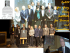More information

### Coherence Network connections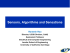More information

### Sparse recovery without incoherence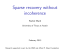More information

### Immunité intracellulaire et la détection des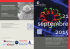More information

### PrimeFilm 1800 AFL Low-Cost 35mm Slide/Film Scanning Through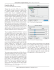More information

### Статья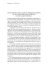More information

### 3. Newsletter Aug - Sep 2015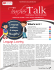More information

### Myers Briggs Type Indicator (MBTI)More information

### Dictionary Optimization for Block-Sparse - Lihi Zelnik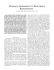However, permission to use this material for any other purposes must be obtained from the IEEE by sending a request to [email protected] The authors are with the Technion - Israel Institut...

More information

### 2007-2008 Ducati Monster S4R/SMore information

### July – December 2014 - The Womanity Foundation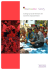More information

### Tivoli Gazebo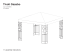More information

### YOUNG`S INTERFERENCE EXPERIMENT: THE LONG AND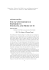More information

### R - imekoMore information

### PDF doc - Adobe Experience Manager 6.2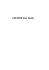More information

### Livelink WCM Server Programming Guide for the WCM Java API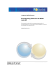More information

### Livelink Integration Manual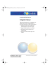More information